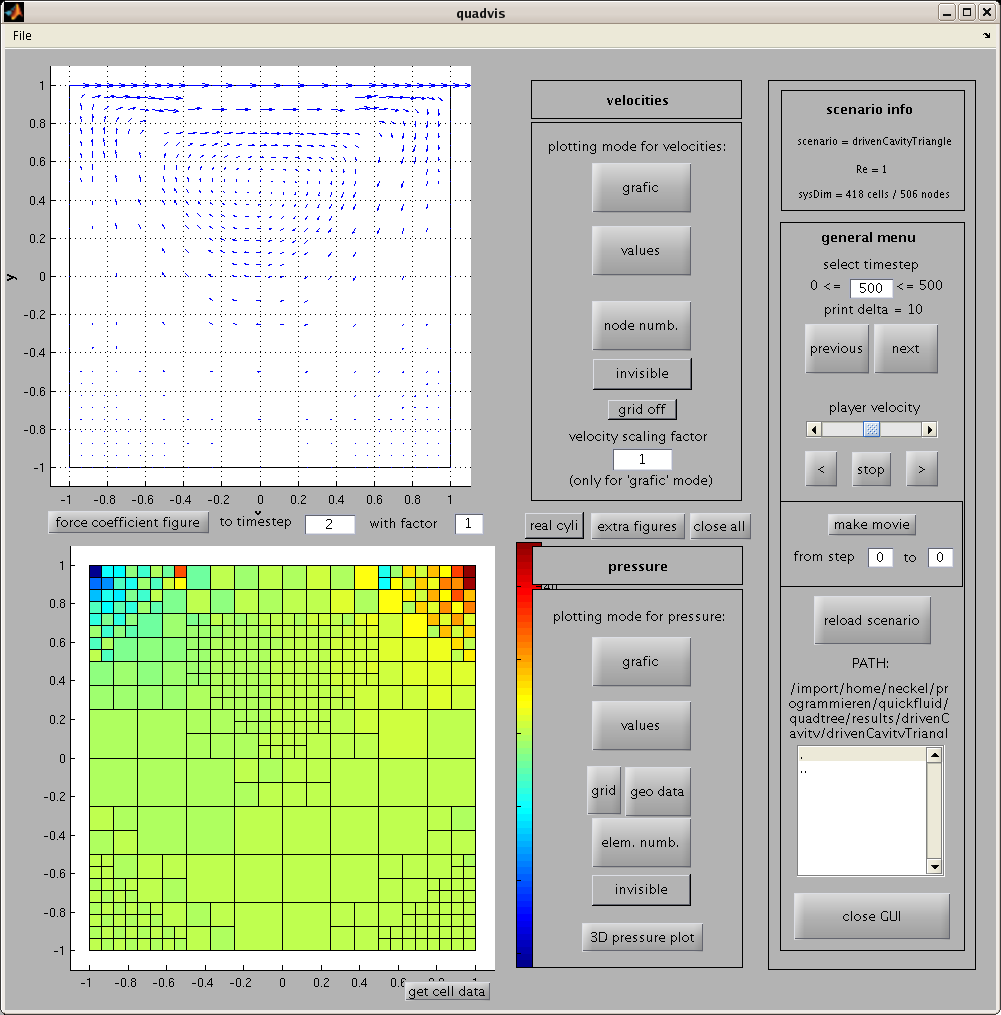[an error occurred while processing this directive]

### Matlab package for the numerical simulation of 2D laminar, incompressible fluid flow on adaptive grids.quadfluid solves the unsteady Navier-Stokes equations in two dimension on adaptive Cartesian grids (quadtree) as a testbed for mathematical and physical methods in the context of flow simulation.
Based on the Chorin projection method, the time discretisation of the momentum equations is done by an explicit Euler method. The discretisation of the space is realised by bilinear elements for the velocities using constant pressure per cell as the corresponding Lagrangeian multiplier for the continuity constraint. In each time step, thus, a linear system of equations (the discrete pressure poisson equation) is solved whose solution is used to update the velocities for the next time step. For the calculation of force values (on obstacles, e.g.), the method of consistent forces is used. Special care (interpolation etc.) is applied whenever coarser and finer grid cells touch each other.Screenshot of the matlab-GUI quadvis.

Via the use of obstacle node lists, arbitrary geometries can be used in a rectangular domain. Built in scenarios are e.g. driven cavity, free channel flow, cylinder channel benchmarks, and the CFD1 benchmark of the Forschergruppe 493 zur Fluid-Struktur-Wechselwirkung.

This is an extension of the version for regular grids: quickfluid.

The program package comes with an easy to use visualisation GUI called quadvis.

A version of this matlab package is available by contacting T. Neckel
T. Neckel, 11.04.2007